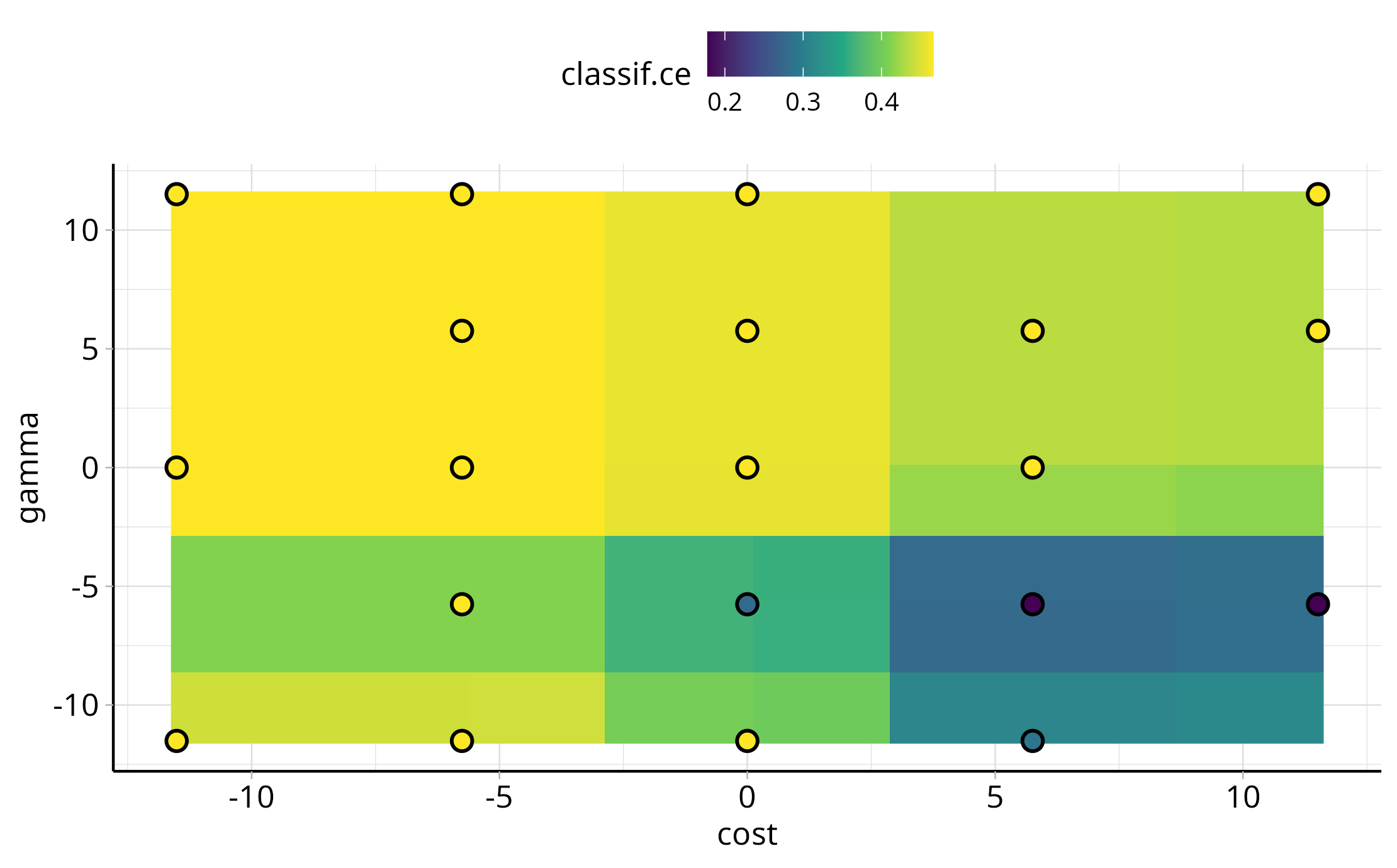Package website: release | dev

mlr3tuning is the hyperparameter optimization package of the mlr3 ecosystem. It features highly configurable search spaces via the paradox package and finds optimal hyperparameter configurations for any mlr3 learner. mlr3tuning works with several optimization algorithms e.g. Random Search, Iterated Racing, Bayesian Optimization (in mlr3mbo) and Hyperband (in mlr3hyperband). Moreover, it can automatically optimize learners and estimate the performance of optimized models with nested resampling. The package is built on the optimization framework bbotk.

## Extension packages

mlr3tuning is extended by the following packages.

• mlr3tuningspaces is a collection of search spaces from scientific articles for commonly used learners.
• mlr3hyperband adds the Hyperband and Successive Halving algorithm.
• mlr3mbo adds Bayesian Optimization methods.

## Resources

There are several sections about hyperparameter optimization in the mlr3book.

The gallery features a collection of case studies and demos about optimization.

The cheatsheet summarizes the most important functions of mlr3tuning.

## Installation

Install the last release from CRAN:

install.packages("mlr3tuning")

Install the development version from GitHub:

remotes::install_github("mlr-org/mlr3tuning")

## Examples

We optimize the cost and gamma hyperparameters of a support vector machine on the Sonar data set.

library("mlr3verse")

learner = lrn("classif.svm",
cost  = to_tune(1e-5, 1e5, logscale = TRUE),
gamma = to_tune(1e-5, 1e5, logscale = TRUE),
type = "C-classification"
)

We construct a tuning instance with the ti() function. The tuning instance describes the tuning problem.

instance = ti(
learner = learner,
resampling = rsmp("cv", folds = 3),
measures = msr("classif.ce"),
terminator = trm("none")
)
instance
## <TuningInstanceSingleCrit>
## * State:  Not optimized
## * Objective: <ObjectiveTuning:classif.svm_on_sonar>
## * Search Space:
##       id    class     lower    upper nlevels
## 1:  cost ParamDbl -11.51293 11.51293     Inf
## 2: gamma ParamDbl -11.51293 11.51293     Inf
## * Terminator: <TerminatorNone>

We select a simple grid search as the optimization algorithm.

tuner = tnr("grid_search", resolution = 5)
tuner
## <TunerGridSearch>: Grid Search
## * Parameters: resolution=5, batch_size=1
## * Parameter classes: ParamLgl, ParamInt, ParamDbl, ParamFct
## * Properties: dependencies, single-crit, multi-crit
## * Packages: mlr3tuning

To start the tuning, we simply pass the tuning instance to the tuner.

tuner$optimize(instance) ## cost gamma learner_param_vals x_domain classif.ce ## 1: 11.51293 -5.756463 <list> <list> 0.1779158 The tuner returns the best hyperparameter configuration and the corresponding measured performance. The archive contains all evaluated hyperparameter configurations. as.data.table(instance$archive)[, .(cost, gamma, classif.ce, batch_nr, resample_result)]
##           cost      gamma classif.ce batch_nr      resample_result
##  1:  11.512925  -5.756463  0.1779158        1 <ResampleResult>
##  2:  11.512925  11.512925  0.4662526        2 <ResampleResult>
##  3:   5.756463   5.756463  0.4662526        3 <ResampleResult>
##  4:  -5.756463  -5.756463  0.4662526        4 <ResampleResult>
##  5: -11.512925   0.000000  0.4662526        5 <ResampleResult>
## ---
## 21: -11.512925  -5.756463  0.4662526       21 <ResampleResult>
## 22:  11.512925   0.000000  0.4662526       22 <ResampleResult>
## 23:   5.756463  11.512925  0.4662526       23 <ResampleResult>
## 24:  11.512925 -11.512925  0.2498965       24 <ResampleResult>
## 25: -11.512925   5.756463  0.4662526       25 <ResampleResult>

The mlr3viz package visualizes tuning results.

library(mlr3viz)

autoplot(instance, type = "surface")We fit a final model with optimized hyperparameters to make predictions on new data.

learner$param_set$values = instance$result_learner_param_vals learner$train(tsk("sonar"))